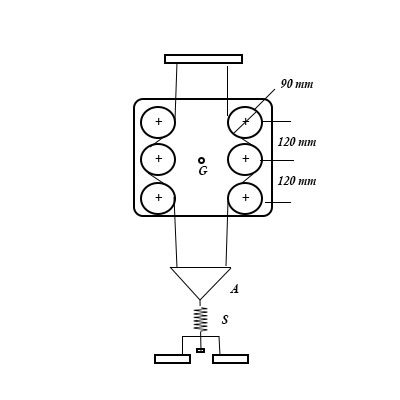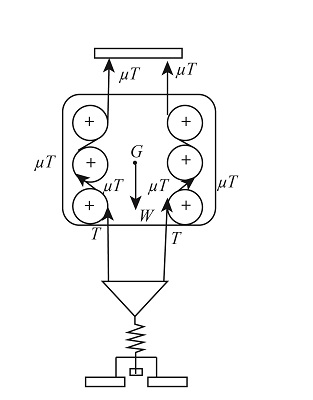# The assembly shown below has a mass of 100 kg with center of mass at G. It is supported by two...

## Question:

The assembly shown below has a mass of {eq}100 kg {/eq} with center of mass at {eq}G {/eq}. It is supported by two wires which pass around the fixed pegs and are kept under equal tensions by the equalizer plate {eq}A {/eq} and the adjustable spring {eq}S {/eq}. Calculate the minimum tension {eq}T {/eq} in the spring which will ensure that the assembly remains suspended as shown. The friction coefficient between the wires and the pegs is {eq}0.3. {/eq}## Friction

When a resisting force acts on an object in contact that are in relative motion with respect to each other is termed as friction. The friction can act in all cases whether it is dry condition or wet condition.

Given data

• Mass of assemble is: {eq}m = 100\;{\rm{kg}} {/eq}
• Coefficient of friction between wire and pegs is: {eq}\mu = 0.3 {/eq}
• Number of pegs on one side is: {eq}n = 3 {/eq}

The figure below shows the free body diagram of assembly.The formula for weight of assembly.

{eq}W = mg {/eq}

Substitute the given values in above formula,

{eq}\begin{align*} W &= 100\;{\rm{kg}} \times 9.81\;{\rm{m/}}{{\rm{s}}^{\rm{2}}}\\ W &= 981\;{\rm{N}} \end{align*} {/eq}

The formula for equilibrium of forces in vertical direction.

{eq}\begin{align*} \sum {{F_y}} &= 0\\ 2n \times \mu T - W &= 0 \end{align*} {/eq}

Here, the tension in the string is {eq}T {/eq}.

Substitute the given values in above formula,

{eq}\begin{align*} 2 \times 3 \times 0.3 \times T - 981\;{\rm{N}} &= 0\\ T &= 545\;{\rm{N}} \end{align*} {/eq}

Thus, the minimum required tension is {eq}545\;{\rm{N}} {/eq}Friction: Definition and Types

from

Chapter 7 / Lesson 5
39K

You experience friction all the time, and you should be glad you do! Friction helps keep stationary objects in place as well as slow moving objects down as they slide across a surface. This lesson identifies what friction is and explains the two ways we find this force on earth.